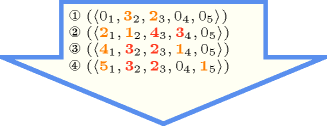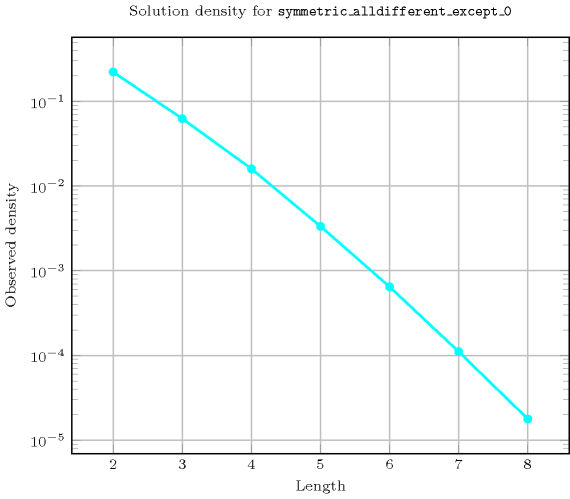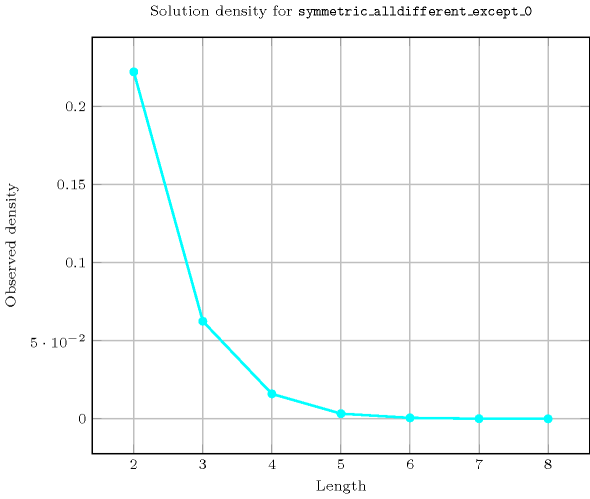## 5.396. symmetric_alldifferent_except_0

Origin
Constraint

$\mathrm{𝚜𝚢𝚖𝚖𝚎𝚝𝚛𝚒𝚌}_\mathrm{𝚊𝚕𝚕𝚍𝚒𝚏𝚏𝚎𝚛𝚎𝚗𝚝}_\mathrm{𝚎𝚡𝚌𝚎𝚙𝚝}_\mathtt{0}\left(\mathrm{𝙽𝙾𝙳𝙴𝚂}\right)$

Synonyms

$\mathrm{𝚜𝚢𝚖𝚖𝚎𝚝𝚛𝚒𝚌}_\mathrm{𝚊𝚕𝚕𝚍𝚒𝚏𝚏}_\mathrm{𝚎𝚡𝚌𝚎𝚙𝚝}_\mathtt{0}$, $\mathrm{𝚜𝚢𝚖𝚖𝚎𝚝𝚛𝚒𝚌}_\mathrm{𝚊𝚕𝚕𝚍𝚒𝚜𝚝𝚒𝚗𝚌𝚝}_\mathrm{𝚎𝚡𝚌𝚎𝚙𝚝}_\mathtt{0}$, $\mathrm{𝚜𝚢𝚖𝚖}_\mathrm{𝚊𝚕𝚕𝚍𝚒𝚏𝚏𝚎𝚛𝚎𝚗𝚝}_\mathrm{𝚎𝚡𝚌𝚎𝚙𝚝}_\mathtt{0}$, $\mathrm{𝚜𝚢𝚖𝚖}_\mathrm{𝚊𝚕𝚕𝚍𝚒𝚏𝚏}_\mathrm{𝚎𝚡𝚌𝚎𝚙𝚝}_\mathtt{0}$, $\mathrm{𝚜𝚢𝚖𝚖}_\mathrm{𝚊𝚕𝚕𝚍𝚒𝚜𝚝𝚒𝚗𝚌𝚝}_\mathrm{𝚎𝚡𝚌𝚎𝚙𝚝}_\mathtt{0}$.

Argument
 $\mathrm{𝙽𝙾𝙳𝙴𝚂}$ $\mathrm{𝚌𝚘𝚕𝚕𝚎𝚌𝚝𝚒𝚘𝚗}\left(\mathrm{𝚒𝚗𝚍𝚎𝚡}-\mathrm{𝚒𝚗𝚝},\mathrm{𝚜𝚞𝚌𝚌}-\mathrm{𝚍𝚟𝚊𝚛}\right)$
Restrictions
 $\mathrm{𝚛𝚎𝚚𝚞𝚒𝚛𝚎𝚍}$$\left(\mathrm{𝙽𝙾𝙳𝙴𝚂},\left[\mathrm{𝚒𝚗𝚍𝚎𝚡},\mathrm{𝚜𝚞𝚌𝚌}\right]\right)$ $\mathrm{𝙽𝙾𝙳𝙴𝚂}.\mathrm{𝚒𝚗𝚍𝚎𝚡}\ge 1$ $\mathrm{𝙽𝙾𝙳𝙴𝚂}.\mathrm{𝚒𝚗𝚍𝚎𝚡}\le |\mathrm{𝙽𝙾𝙳𝙴𝚂}|$ $\mathrm{𝚍𝚒𝚜𝚝𝚒𝚗𝚌𝚝}$$\left(\mathrm{𝙽𝙾𝙳𝙴𝚂},\mathrm{𝚒𝚗𝚍𝚎𝚡}\right)$ $\mathrm{𝙽𝙾𝙳𝙴𝚂}.\mathrm{𝚜𝚞𝚌𝚌}\ge 0$ $\mathrm{𝙽𝙾𝙳𝙴𝚂}.\mathrm{𝚜𝚞𝚌𝚌}\le |\mathrm{𝙽𝙾𝙳𝙴𝚂}|$
Purpose

Enforce the following three conditions:

1. $\forall i\in \left[1,|\mathrm{𝙽𝙾𝙳𝙴𝚂}|\right]$, $\forall j\in \left[1,|\mathrm{𝙽𝙾𝙳𝙴𝚂}|\right]$, ($j\ne i$): $\mathrm{𝙽𝙾𝙳𝙴𝚂}\left[i\right].\mathrm{𝚜𝚞𝚌𝚌}=0\vee \mathrm{𝙽𝙾𝙳𝙴𝚂}\left[j\right].\mathrm{𝚜𝚞𝚌𝚌}=0\vee \mathrm{𝙽𝙾𝙳𝙴𝚂}\left[i\right].\mathrm{𝚜𝚞𝚌𝚌}\ne \mathrm{𝙽𝙾𝙳𝙴𝚂}\left[j\right].\mathrm{𝚜𝚞𝚌𝚌}$.

2. $\forall i\in \left[1,|\mathrm{𝙽𝙾𝙳𝙴𝚂}|\right]:\mathrm{𝙽𝙾𝙳𝙴𝚂}\left[i\right].\mathrm{𝚜𝚞𝚌𝚌}\ne i$.

3. $\mathrm{𝙽𝙾𝙳𝙴𝚂}\left[i\right].\mathrm{𝚜𝚞𝚌𝚌}=j\wedge j\ne i\wedge j\ne 0⇔\mathrm{𝙽𝙾𝙳𝙴𝚂}\left[j\right].\mathrm{𝚜𝚞𝚌𝚌}=i\wedge i\ne j\wedge i\ne 0$.

Example
$\left(\begin{array}{c}〈\begin{array}{cc}\mathrm{𝚒𝚗𝚍𝚎𝚡}-1\hfill & \mathrm{𝚜𝚞𝚌𝚌}-3,\hfill \\ \mathrm{𝚒𝚗𝚍𝚎𝚡}-2\hfill & \mathrm{𝚜𝚞𝚌𝚌}-0,\hfill \\ \mathrm{𝚒𝚗𝚍𝚎𝚡}-3\hfill & \mathrm{𝚜𝚞𝚌𝚌}-1,\hfill \\ \mathrm{𝚒𝚗𝚍𝚎𝚡}-4\hfill & \mathrm{𝚜𝚞𝚌𝚌}-0\hfill \end{array}〉\hfill \end{array}\right)$

The $\mathrm{𝚜𝚢𝚖𝚖𝚎𝚝𝚛𝚒𝚌}_\mathrm{𝚊𝚕𝚕𝚍𝚒𝚏𝚏𝚎𝚛𝚎𝚗𝚝}_\mathrm{𝚎𝚡𝚌𝚎𝚙𝚝}_\mathtt{0}$ constraint holds since:

• $\mathrm{𝙽𝙾𝙳𝙴𝚂}\left[1\right].\mathrm{𝚜𝚞𝚌𝚌}=3⇔\mathrm{𝙽𝙾𝙳𝙴𝚂}\left[3\right].\mathrm{𝚜𝚞𝚌𝚌}=1$,

• $\mathrm{𝙽𝙾𝙳𝙴𝚂}\left[2\right].\mathrm{𝚜𝚞𝚌𝚌}=0$ and value 2 is not assigned to any variable.

• $\mathrm{𝙽𝙾𝙳𝙴𝚂}\left[4\right].\mathrm{𝚜𝚞𝚌𝚌}=0$ and value 4 is not assigned to any variable.

Given 3 successor variables that have to be assigned a value in interval $\left[0,3\right]$, the solutions to the $\mathrm{𝚜𝚢𝚖𝚖𝚎𝚝𝚛𝚒𝚌}_\mathrm{𝚊𝚕𝚕𝚍𝚒𝚏𝚏𝚎𝚛𝚎𝚗𝚝}_\mathrm{𝚎𝚡𝚌𝚎𝚙𝚝}_\mathtt{0}$ $\left(〈\mathrm{𝚒𝚗𝚍𝚎𝚡}-1\mathrm{𝚜𝚞𝚌𝚌}-{s}_{1},\mathrm{𝚒𝚗𝚍𝚎𝚡}-2\mathrm{𝚜𝚞𝚌𝚌}-{s}_{2},\mathrm{𝚒𝚗𝚍𝚎𝚡}-3\mathrm{𝚜𝚞𝚌𝚌}-{s}_{3}〉\right)$ constraint are $〈10,20,30〉$, $〈10,23,32〉$, $〈12,21,30〉$, and $〈13,20,31〉$.

Given 4 successor variables that have to be assigned a value in interval $\left[0,3\right]$, the solutions to the $\mathrm{𝚜𝚢𝚖𝚖𝚎𝚝𝚛𝚒𝚌}_\mathrm{𝚊𝚕𝚕𝚍𝚒𝚏𝚏𝚎𝚛𝚎𝚗𝚝}_\mathrm{𝚎𝚡𝚌𝚎𝚙𝚝}_\mathtt{0}$ $\left(〈\mathrm{𝚒𝚗𝚍𝚎𝚡}-1\mathrm{𝚜𝚞𝚌𝚌}-{s}_{1},\mathrm{𝚒𝚗𝚍𝚎𝚡}-2\mathrm{𝚜𝚞𝚌𝚌}-{s}_{2},\mathrm{𝚒𝚗𝚍𝚎𝚡}-3\mathrm{𝚜𝚞𝚌𝚌}-{s}_{3},\mathrm{𝚒𝚗𝚍𝚎𝚡}-4\mathrm{𝚜𝚞𝚌𝚌}-{s}_{4}〉\right)$ constraint are $〈10,20,30,40〉$, $〈10,20,34,43〉$, $〈10,23,32,40〉$, $〈10,24,30,42〉$, $〈12,21,30,40〉$, $〈12,21,34,43〉$, $〈13,20,31,40〉$, $〈13,24,31,42〉$, $〈14,20,30,41〉$, $〈14,23,32,41〉$.

All solutions

Figure 5.396.1 gives all solutions to the following non ground instance of the $\mathrm{𝚜𝚢𝚖𝚖𝚎𝚝𝚛𝚒𝚌}_\mathrm{𝚊𝚕𝚕𝚍𝚒𝚏𝚏𝚎𝚛𝚎𝚗𝚝}_\mathrm{𝚎𝚡𝚌𝚎𝚙𝚝}_\mathtt{0}$ constraint: ${S}_{1}\in \left[0..5\right]$, ${S}_{2}\in \left[1..3\right]$, ${S}_{3}\in \left[1..4\right]$, ${S}_{4}\in \left[0..3\right]$, ${S}_{5}\in \left[0..2\right]$, $\mathrm{𝚜𝚢𝚖𝚖𝚎𝚝𝚛𝚒𝚌}_\mathrm{𝚊𝚕𝚕𝚍𝚒𝚏𝚏𝚎𝚛𝚎𝚗𝚝}_\mathrm{𝚎𝚡𝚌𝚎𝚙𝚝}_\mathtt{0}$$\left(〈1{S}_{1},2{S}_{2},3{S}_{3},4{S}_{4},5{S}_{5}〉\right)$.

##### Figure 5.396.1. All solutions corresponding to the non ground example of the $\mathrm{𝚜𝚢𝚖𝚖𝚎𝚝𝚛𝚒𝚌}_\mathrm{𝚊𝚕𝚕𝚍𝚒𝚏𝚏𝚎𝚛𝚎𝚗𝚝}_\mathrm{𝚎𝚡𝚌𝚎𝚙𝚝}_\mathtt{0}$ constraint of the All solutions slot (the $\mathrm{𝚒𝚗𝚍𝚎𝚡}$ attribute is displayed as indices of the $\mathrm{𝚜𝚞𝚌𝚌}$ attribute)Typical
 $|\mathrm{𝙽𝙾𝙳𝙴𝚂}|\ge 4$ $\mathrm{𝚖𝚒𝚗𝚟𝚊𝚕}$$\left(\mathrm{𝙽𝙾𝙳𝙴𝚂}.\mathrm{𝚜𝚞𝚌𝚌}\right)=0$ $\mathrm{𝚖𝚊𝚡𝚟𝚊𝚕}$$\left(\mathrm{𝙽𝙾𝙳𝙴𝚂}.\mathrm{𝚜𝚞𝚌𝚌}\right)>0$
Symmetry

Items of $\mathrm{𝙽𝙾𝙳𝙴𝚂}$ are permutable.

Usage

Within the context of sport scheduling, $\mathrm{𝙽𝙾𝙳𝙴𝚂}\left[i\right].\mathrm{𝚜𝚞𝚌𝚌}=j$ ($i\ne 0,j\ne 0,i\ne j$) is interpreted as the fact that team $i$ plays against team $j$, while $\mathrm{𝙽𝙾𝙳𝙴𝚂}\left[i\right].\mathrm{𝚜𝚞𝚌𝚌}=0$ ($i\ne 0$) is interpreted as the fact that team $i$ does not play at all.

Algorithm

An arc-consistency filtering algorithm for the $\mathrm{𝚜𝚢𝚖𝚖𝚎𝚝𝚛𝚒𝚌}_\mathrm{𝚊𝚕𝚕𝚍𝚒𝚏𝚏𝚎𝚛𝚎𝚗𝚝}_\mathrm{𝚎𝚡𝚌𝚎𝚙𝚝}_\mathtt{0}$ constraint is described in [Cymer13], [CymerPhD13]. The algorithm is based on the following facts:

• First, one can map solutions to the $\mathrm{𝚜𝚢𝚖𝚖𝚎𝚝𝚛𝚒𝚌}_\mathrm{𝚊𝚕𝚕𝚍𝚒𝚏𝚏𝚎𝚛𝚎𝚗𝚝}_\mathrm{𝚎𝚡𝚌𝚎𝚙𝚝}_\mathtt{0}$ constraint to perfect $\left(g,f\right)$-matchings in a non-bipartite graph derived from the domain of the variables of the constraint where $g\left(x\right)=0$, $f\left(x\right)=1$ for vertices $x$ which have 0 in their domain, and $g\left(x\right)=f\left(x\right)=1$ for all the remaining vertices. A perfect $\left(g,f\right)$-matching $ℳ$ of a graph is a subset of edges such that every vertex $x$ is incident with the number of edges in $ℳ$ between $g\left(x\right)$ and $f\left(x\right)$.

• Second, Gallai-Edmonds decomposition [Gallai63], [Edmonds65] allows to find out all edges that do not belong to any perfect $\left(g,f\right)$-matchings, and therefore prune the corresponding variables.

Counting
 Length ($n$) 2 3 4 5 6 7 8 Solutions 2 4 10 26 76 232 764

Number of solutions for $\mathrm{𝚜𝚢𝚖𝚖𝚎𝚝𝚛𝚒𝚌}_\mathrm{𝚊𝚕𝚕𝚍𝚒𝚏𝚏𝚎𝚛𝚎𝚗𝚝}_\mathrm{𝚎𝚡𝚌𝚎𝚙𝚝}_\mathtt{0}$: domains $0..n$$\mathrm{𝚜𝚢𝚖𝚖𝚎𝚝𝚛𝚒𝚌}_\mathrm{𝚊𝚕𝚕𝚍𝚒𝚏𝚏𝚎𝚛𝚎𝚗𝚝}_\mathrm{𝚎𝚡𝚌𝚎𝚙𝚝}_\mathtt{0}\left(\mathrm{𝙽𝙾𝙳𝙴𝚂}\right)$
implies $\mathrm{𝚊𝚕𝚕𝚍𝚒𝚏𝚏𝚎𝚛𝚎𝚗𝚝}_\mathrm{𝚎𝚡𝚌𝚎𝚙𝚝}_\mathtt{0}$$\left(\mathrm{𝚅𝙰𝚁𝙸𝙰𝙱𝙻𝙴𝚂}:\mathrm{𝙽𝙾𝙳𝙴𝚂}\right)$.